# Class 10 Maths Important Questions for Statistics

Given below are the Class 10 Maths Important and extra Questions for Statistics
(a) Fill in the blank's
(b) True and false
(c) Multiple choice questions
(d) Match the column

## Fill in the blank

Question 1
(a) The mean of 50 numbers is 18, the new mean will be _____ if each observation is increased by 4. ( 22/24/20)
(b) The sum of deviations of a set of values {a,b,c,d,e,f,g.h.i ______} n items  measured from 26 is -10 and the sum of deviations of the values from 20 is 50. The value of n is ______(10/12/9) And mean of the items is _______. ( 19/18/17/25)
(c) For a given data with 110 observations the ‘less than ogive’ and the ‘more then ogive’ intersect at (18, 20). The median of the data is _____. (18/20/19)
(d) The curve drawn by taking upper limits along x-axis and cumulative frequency along y-axis is _______.( less than ogive /more than ogive)
(e) The mean of five numbers is 40. If one number is excluded, their mean becomes 28. The excluded number is _________. (68 / 88)
(f) The modal class of the grouped size frequency table given below is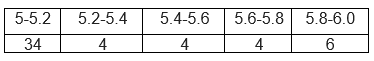Solution
(a) 24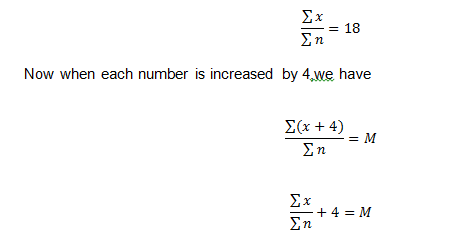So M=22
(b) 10,25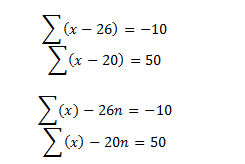Subtracting ,we get
-6n=-60 => n=10
So $\sum x=250$
Mean=250/10=25
(c) 18.    Median is the point of intersection of ogive curves
(d) less than ogive curve
(e) 88
Sum of five number=5Xmean=200
Sum of four number=4Xmean=112
Subtracting, we get the number=88
(f) Modal class is 5-5.2

## True or False statement

Question 2
The following shows the class interval and respective frequency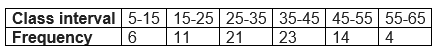(a) The mean is 33
(b) The modal class is 35-45
(c) The mode is 34
(d) The Frequency for less than is 35 is 38
(e)  The median is
Solution
Lets find the mean value first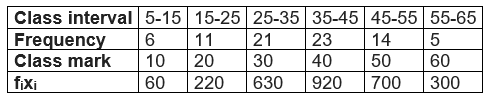Mean is given by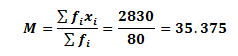The class having highest frequency is called the Modal class, so Modal class is 35-45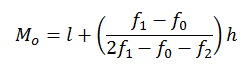Where
l = lower limit of the modal class,
h = size of the class interval (assuming all class sizes to be equal),
f1 = frequency of the modal class,
f0 = frequency of the class preceding the modal class,
f2 = frequency of the class succeeding the modal class.
Now l=35 h=10 f1=23 f0=21 and f2=14
So substituting all the values we get
0=36.81
Now
3 Median=Mode +2 Mean
So median= (Mode +2 Mean)/3=35.853
(a) False. I
(b) True
(c) False
(d) True.
(e) False

## Multiple choice Questions

Question 3
While computing mean of grouped data, we assume that the frequencies are
(a)centred at the upper limits of the classes
(b)centred at the lower limits of the classes
(c)centred at the class-marks of the classes
(d)evenly distributed over all the classes
Solution (c)

Question 4
Which of the following is the measure of central tendency?
(a) Mean
(b)Mode
(c)Median
(d) Range
Solution (a) ,(b),(c)

Question 5
For drawing a frequency polygon of a continuous frequency distribution, we plot the Points whose ordinates are the frequencies of the respective classes and abscissae are respectively :
(a) class marks of the classes
(b) upper limits of preceding classes
(c) lower limits of the classes
(d) upper limits of the classes
Solution (a)

Question 6
Find the mean of 32 numbers given mean of ten of them is 12 and the mean of other 20 is 9. And last 2 number is 10
(a)10
(b) 12
(c) 13
(d) 14
Solution (a)
Mean of 10 number =12
Sum of these 10 numbers =120
Mean of 20 number =9
Sum of these 20 numbers =180
mean of 2 number =10
Sum of these 2 numbers =20
Mean of 32 number= Sum of all number/32=(120+180+20)/32=10

Question 7
The median and mean of the first 10 natural numbers.
(a) 5.5,5.5
(b) 5.5,6
(c) 5,6
(d) None of these
Solution (a)
Mean =5.5
Median is  mean of 5 and 6 th term, So 5

Question 8
Anand says that the median of 3, 14, 19, 20, 11 is 19. What doesn’t the Anand understand about finding the median?
(a) The dataset should be ascending order
(b) Highest no in the dataset is the median
(c) Average of lowest and highest is the median
(d) None of these
Solution (a)

Question 9
The following observations are arranged in ascending order :
20, 23, 42, 53, x, x + 2, 70, 75, 82, 96
If the median is 63, find the value of x.
(a) 62
(b) 64
(c) 60
(d) None of these
Solution (a)
Median is mean of 5 and 6 term
So x+1=63
x=62

Question 10
The mean of 20 observations was 60. It was detected on rechecking that the value of 125 was wrongly copied as 25 for computation of mean. Find the correct mean
(a) 67
(b) 66
(c) 65
(d) None of the above
Solution
Let x be the sum of observation of 19 numbers leaving 125,
Then
$x+25=20 \times 60=1200$
Now
$x+125=20 \times y=20y$
Subtracting
$125-25=20y-1200$
$20y=1300$
y=65

## Match the column

Question 11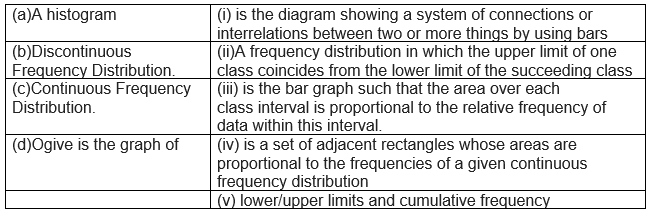Question 11
Compute the Median for the given data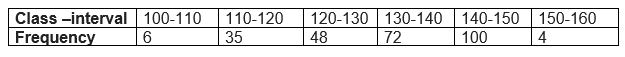Solution
First calculate the Class mark and cumulative frequency of the data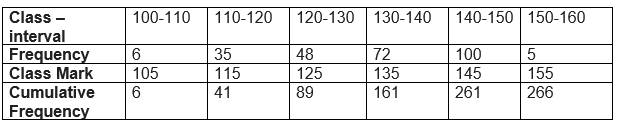We have N=266, So N/2=133,Cummulative frequency first greater than  133,lies in class 130-140
So median class is 130-140
Now
Median is calculated as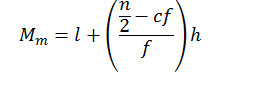Where
l = lower limit of median class,
n = number of observations,
cf = cumulative frequency of class preceding the median class,
f = frequency of median class,
h = class size (assuming class size to be equal)
Here l=130 n=266 cf=89 f=72 h=10
Substituting these
M=  130+(133-89)x10/72=136.11

• Notes
• Assignments
• NCERT SolutionsGo back to Class 10 Main Page using below links

### Practice Question

Question 1 What is $1 - \sqrt {3}$ ?
A) Non terminating repeating
B) Non terminating non repeating
C) Terminating
D) None of the above
Question 2 The volume of the largest right circular cone that can be cut out from a cube of edge 4.2 cm is?
A) 19.4 cm3
B) 12 cm3
C) 78.6 cm3
D) 58.2 cm3
Question 3 The sum of the first three terms of an AP is 33. If the product of the first and the third term exceeds the second term by 29, the AP is ?
A) 2 ,21,11
B) 1,10,19
C) -1 ,8,17
D) 2 ,11,20